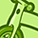# QlikView App Dev

Discussion Board for collaboration related to QlikView App Development.

Announcements
Join this live chat April 6, 10AM EST - QlikView to Qlik Sense REGISTER
cancel
Showing results for
Did you mean:Creator III

## Static Dimension in Chart

Hi All,

I have one Qlik Dashboard where i have more than 150 KPIs'. in that like few metrics are Percentage , few or numerical like that so for that i created a Flag where all Percent metrics will come under one and numerical metrics will come under different like below

Here 1 is all Percentage metrics and Second is Numerical.

IF(Metric='Customer Utilization' OR
Metric='Absence' OR
Metric='Investment Utilization' OR
Metric='Minimum Time Submitted (MTS)' OR
Metric='Pre-Sales Utilization'

, 1,

IF(Metric='Activity Hours (K)' OR
Metric='Portfolio Hours Global (K)'
, 2,

3)) AS Metric_Flag,

So when i comes to front end in Chart Expression i have given as below

If ( MAX ( {<ScenarioLabel={'Actuals'},Scope={'Global'}>} Metric_Flag ) = 1,

num ( SUM ({<ScenarioLabel={'Actuals'},Scope={'Global'}>} NumValue) /
SUM ({<ScenarioLabel={'Actuals'},Scope={'Global'}>} DenValue), '0.#%') ,

If ( MAX ( {<ScenarioLabel={'Actuals'},Scope={'Global'}>} Metric_Flag ) = 2,

num ( SUM ({<ScenarioLabel={'Actuals'},Scope={'Global'}>} NumValue),'##,###.0'),

Issue is

But i have different filters like month, Country , etc   so if some one select some country or Month from filter and that country or Month has no Data in backend Fact table  . My Metrics is Disappearing in  Chart Pivot Dimension which it should not.

the only point is my Metrics Dimension should be static  in Chart Pivot Dimension, whether it has data or not it should not go off.

how can we do that ?

1 Solution

Accepted SolutionsPartner

Try this:What I did is to add in a line of expression, excluding all dimensions and multiply with 0.
So that the new line of expression did not affect the original expression.

Next in presentation, uncheck suppress zero values.

Refer qvw attached.

Thanks and regards,
Arthur Fong

9 RepliesPartner

You have a sample app?Creator III
Author

Hi,

I am attaching the app.

in the attached app basically , if you select GIC in Delivery Group filter my metrics are going off.

what ever the scenario what ever dimension i select like month or any geo my metrics list should be static it should not go off. that's what i need .Partner

Try this:What I did is to add in a line of expression, excluding all dimensions and multiply with 0.
So that the new line of expression did not affect the original expression.

Next in presentation, uncheck suppress zero values.

Refer qvw attached.

Thanks and regards,
Arthur FongContributor III

Hi ,

Thank You.

it works in that way, but again format changes ,

bec in that \$(vLESQBRNEW) i have wrote multiple flags like metrics with flag 1 contains all percentage metrics , metrics with flag 2 contains  numerical number and so on.

so is it some thing we can add to  below  expression directly.  same below expression is nothing but \$(vLESQBRNEW) this variable same you can get it from app in variable Section.

instead of writing below as format changes ,, can we add to variable expression directly ?

\$(vLESQBRNEW)
+
(SUM ({1<ScenarioLabel={'Actuals'},Month={">=\$(vMinMonth) <=\$(vGetMaxQuarterMonthFinal)"}>} NumValue)*0)

Expression :

IF ( MAX( {\$<FY_QM={'Threshold'},Scope={'LES'}, QBR={'Primary (QBR)'},FYear={'Threshold'},FY_MonthName={'Threshold'},WW=,Region=, Geography=, SubGeo1=, SubGeo2=, Country=,QuarterID=>} TargetFlag) = 1,
IF( MIN({\$<FY_QM={'Threshold'},Scope={'LES'},QBR={'Primary (QBR)'},WW=,Region=, Geography=, SubGeo1=, SubGeo2=, Country=,Area=,Region_memberid_label={\$(vUnqGeography)},QBR=,Month=,FY_Month=,FY_QM=,FY_Quarter=,FYear=,QuarterID=>} NumValue)=0,'Dir',
MAXSTRING({\$<FY_QM={'Threshold'},Scope={'LES'},QBR={'Primary (QBR)'},WW=,Region=, Geography=, SubGeo1=, SubGeo2=, Country=,Area=,
Region_memberid_label={\$(vUnqGeography)},Month=,FY_Month=,FY_QM=,FY_Quarter=,FYear=,QuarterID=>} NumValue) ),

If ( MAX ( {<ScenarioLabel={'Actuals'},Scope={'LES'}>} LESMetric_Flag ) = 1,

IF(GetFieldSelections(Geography)>0 OR GetFieldSelections(SubGeo1)>0 OR GetFieldSelections(SubGeo2)>0 OR GetFieldSelections(Country)>0 OR GetFieldSelections(Area)>0 ,

num ( SUM ({<ScenarioLabel={'Actuals'},Scope={'LES'},Month={">=\$(vMinMonth) <=\$(vGetMaxQuarterMonthFinal)"}>} NumValue) /
SUM ({<ScenarioLabel={'Actuals'},Scope={'LES'},Month={">=\$(vMinMonth) <=\$(vGetMaxQuarterMonthFinal)"}>} DenValue), '0.#%'),

num ( SUM ({<ScenarioLabel={'Actuals'},WW={'WW'},Scope={'LES'},Month={">=\$(vMinMonth) <=\$(vGetMaxQuarterMonthFinal)"}>} NumValue) /
SUM ({<ScenarioLabel={'Actuals'},WW={'WW'},Scope={'LES'},Month={">=\$(vMinMonth) <=\$(vGetMaxQuarterMonthFinal)"}>} DenValue), '0.#%')

),

If ( MAX ( {<ScenarioLabel={'Actuals'},Scope={'LES'}>} LESMetric_Flag ) = 2,

IF(GetFieldSelections(Geography)>0 OR GetFieldSelections(SubGeo1)>0 OR GetFieldSelections(SubGeo2)>0 OR GetFieldSelections(Country)>0 OR GetFieldSelections(Area)>0 ,

num ( SUM ({<ScenarioLabel={'Actuals'},Scope={'LES'},Month={">=\$(vMinMonth) <=\$(vGetMaxQuarterMonthFinal)"}>} NumValue),'##,###.0'),

num ( SUM ({<ScenarioLabel={'Actuals'},WW={'WW'},Scope={'LES'},Month={">=\$(vMinMonth) <=\$(vGetMaxQuarterMonthFinal)"}>} NumValue),'##,###.0')

),

If ( MAX ( {<ScenarioLabel={'Actuals'},Scope={'LES'}>} LESMetric_Flag ) = 3,

//IF(WW='WW',num ( SUM ({<ScenarioLabel={'Actuals'},Quarter={\$(vFYQ_5Final)},Scope={'LES'}>} NumValue),'#,##0' ),
num ( SUM ({<ScenarioLabel={'Actuals'},Scope={'LES'},Month={">=\$(vMinMonth) <=\$(vGetMaxQuarterMonthFinal)"}>} NumValue),'#,##0' ),

If ( MAX ( {<ScenarioLabel={'Actuals'},Scope={'LES'}>} LESMetric_Flag ) = 4,

num ( FABS(SUM ({<ScenarioLabel={'Actuals'},Scope={'LES'},SubMetric={'COD'},Metric={'CoD as % Revenue -'},Month={">=\$(vMinMonth) <=\$(vGetMaxQuarterMonthFinal)"}>} NumValue)) /

FABS(SUM ({<ScenarioLabel={'Actuals'},Scope={'LES'},SubMetric={'Rev'},Metric={'CoD as % Revenue -'},Month={">=\$(vMinMonth) <=\$(vGetMaxQuarterMonthFinal)"}>} NumValue))

, '0.#%;0.#%'),

If ( MAX ( {<ScenarioLabel={'Actuals'},Scope={'LES'}>} LESMetric_Flag ) = 5,

IF(GetFieldSelections(Geography)>0 OR GetFieldSelections(SubGeo1)>0 OR GetFieldSelections(SubGeo2)>0 OR GetFieldSelections(Country)>0 OR GetFieldSelections(Area)>0 ,

SUM ({<ScenarioLabel={'Actuals'},Scope={'LES'},Month={">=\$(vMinMonth) <=\$(vGetMaxQuarterMonthFinal)"}>} NumValue) ,

SUM ({<ScenarioLabel={'Actuals'},WW={'WW'},Scope={'LES'},Month={">=\$(vMinMonth) <=\$(vGetMaxQuarterMonthFinal)"}>} NumValue)

),

If ( MAX ( {<ScenarioLabel={'Actuals'},Scope={'LES'}>} LESMetric_Flag ) = 6,

IF(GetFieldSelections(Geography)>0 OR GetFieldSelections(SubGeo1)>0 OR GetFieldSelections(SubGeo2)>0 OR GetFieldSelections(Country)>0 OR GetFieldSelections(Area)>0 ,

//Num(Avg(Aggr(Avg({\$<Month={">=\$(vMinMonth) <=\$(vGetMaxQuarterMonthFinal)"}>}[E2E Deployment TAT]), [E2ETATPROJECTID])),'#,##0' ),

Num(Avg({\$<Month={">=\$(vMinMonth) <=\$(vGetMaxQuarterMonthFinal)"}>}[NumValue]),'#,##0' ) ,

Num(Avg({\$<WW={'WW'},Month={">=\$(vMinMonth) <=\$(vGetMaxQuarterMonthFinal)"}>}[NumValue]),'#,##0' )

),

)))))
)

)Partner
Sure you can.Contributor III

Hi,

Can add for one flag in my expression , i am getting some error.Contributor III

Can You add to above expression please for one Flag of expression when i tried that i am getting error or none of the values i am getting.Partner

Try dividing your expressions into parts and check which part is the error coming from.Contributor III

HI

Yes, it works. thank you.